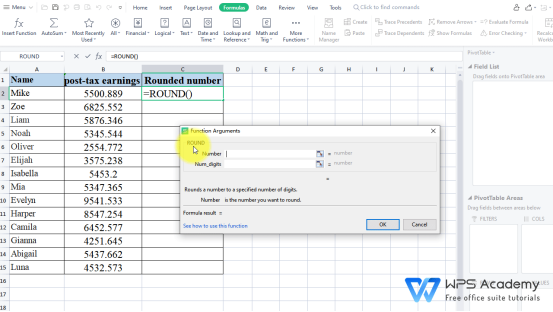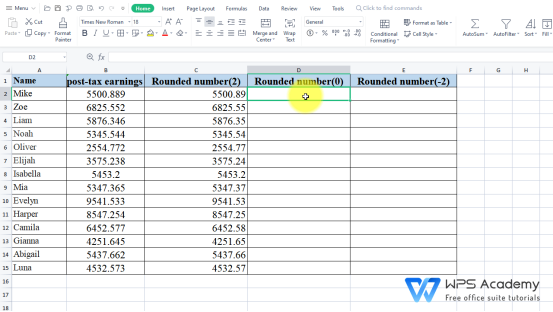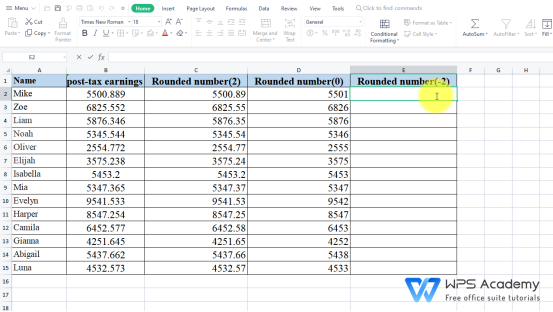# The ROUND function in Excel

December 13, 2021
0.1161 Views

A free Office suite fully compatible with Microsoft Office

WPS Spreadsheet could be an alternative to Microsoft Office Excel. It includes 100's of built-in formulas, pivot tables, and more.

· Description:

In our work and study, we can round off decimals with the help of ROUND function in WPS Spreadsheet.

· Syntax:

ROUND(number, num_digits)

· Argument:

Number – The numerical value to be rounded.

Num_digits – The total number of digits to round the number to.

If the Num_digits is greater than 0, then the number will be rounded to the specified number of Num_digits.

If the Num_digits equals 0, then the number will be rounded to the nearest integer.

If the Num_digits is less than 0, the number will be rounded to the corresponding digit to the left of the decimal point.

· Example:

2. Select the areas that contain the data, then head to the Formula tab > Insert Function. In the pop-up dialog, enter ROUND in the edit box.

3. In theFunction Argument window,enter B2 at Number, and enter 2 at Num_digits, which means we want to two places to the right of the decimal for the value in B2, and the number is going to be rounded up to 5500.89.

4. Click OK, then hove over cell C2, if you want to fill the remaining cells in this column, double-click the plus sign (+) in the bottom right-hand corner.5. To better illustrate this function, let's add two more columns to elaborate on other cases for Num_digits, repeat the above operation.

Rounded number(0):Rounded number(-2):